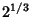## Cube Duplication

Also called the Delian Problem or Duplication of the Cube. A classical problem of antiquity which, given the Edge of a Cube, requires a second Cube to be constructed having double the Volume of the first using only a Straightedge and Compass.

Under these restrictions, the problem cannot be solved because the Delian Constant(the required Ratio of sides of the original Cube and that to be constructed) is not a Euclidean Number. The problem can be solved, however, using a Neusis Construction.

See also Alhazen's Billiard Problem, Compass, Cube, Delian Constant, Geometric Problems of Antiquity, Neusis Construction, Straightedge

References

Ball, W. W. R. and Coxeter, H. S. M. Mathematical Recreations and Essays, 13th ed. New York: Dover, pp. 93-94, 1987.

Conway, J. H. and Guy, R. K. The Book of Numbers. New York: Springer-Verlag, pp. 190-191, 1996.

Courant, R. and Robbins, H. ``Doubling the Cube'' and ``A Classical Construction for Doubling the Cube.'' §3.3.1 and 3.5.1 in What is Mathematics?: An Elementary Approach to Ideas and Methods, 2nd ed. Oxford, England: Oxford University Press, pp. 134-135 and 146, 1996.

Dörrie, H. ``The Delian Cube-Doubling Problem.'' §35 in 100 Great Problems of Elementary Mathematics: Their History and Solutions. New York: Dover, pp. 170-172, 1965.